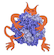IMP  2.4.0 The Integrative Modeling Platform
kernel/DerivativeAccumulator.h
Go to the documentation of this file.
1 /**
2  * \file IMP/kernel/DerivativeAccumulator.h
3  * \brief Class for adding derivatives from
4  * restraints to the model.
5  *
7  *
8  */
9
10 #ifndef IMPKERNEL_DERIVATIVE_ACCUMULATOR_H
11 #define IMPKERNEL_DERIVATIVE_ACCUMULATOR_H
12
13 #include <IMP/kernel/kernel_config.h>
15 #include <IMP/base/check_macros.h>
16 #include <IMP/base/value_macros.h>
17 #include <IMP/base/math.h>
18 #include <IMP/base/exception.h>
19
20 IMPKERNEL_BEGIN_NAMESPACE
21
22 //! Class for adding derivatives from restraints to the model.
23 /** This class was created so that restraints can be weighted using
24  a RestraintSet and that the derivatives would be scaled appropriately */
25 class IMPKERNELEXPORT DerivativeAccumulator {
26  public:
27  IMP_CXX11_DEFAULT_COPY_CONSTRUCTOR(DerivativeAccumulator);
28  //! the weight is one by default
29  DerivativeAccumulator(double weight = 1.0) : weight_(weight) {}
30
31  //! The weight is multiplied by the new weight
32  DerivativeAccumulator(const DerivativeAccumulator &copy, double weight)
33  : weight_(copy.weight_ * weight) {}
34
35  //! Scale a value appropriately.
36  /** \param[in] value Value to add to the float attribute derivative.
37  */
38  double operator()(const double value) const {
39  IMP_INTERNAL_CHECK(!base::isnan(value), "Can't set derivative to NaN.");
40  return value * weight_;
41  }
42  double get_weight() const { return weight_; }
44
45  private:
46  double weight_;
47 };
48
50
51 IMPKERNEL_END_NAMESPACE
52
53 #endif /* IMPKERNEL_DERIVATIVE_ACCUMULATOR_H */
Declare an efficient stl-compatible map.
Class for adding derivatives from restraints to the model.
#define IMP_SHOWABLE_INLINE(Name, how_to_show)
Declare the methods needed by an object that can be printed.
DerivativeAccumulator(const DerivativeAccumulator &copy, double weight)
The weight is multiplied by the new weight.
DerivativeAccumulator(double weight=1.0)
the weight is one by default
Exception definitions and assertions.
double operator()(const double value) const
Scale a value appropriately.
#define IMP_INTERNAL_CHECK(expr, message)
An assertion to check for internal errors in IMP. An IMP::ErrorException will be thrown.
Definition: check_macros.h:141
#define IMP_VALUES(Name, PluralName)
Define the type for storing sets of values.
Definition: value_macros.h:23
bool isnan(const T &a)
Return true if a number is NaN.
Definition: math.h:24
Exception definitions and assertions.
Various general useful macros for IMP.
Various general useful macros for IMP.